## Excel Formula: Check if a cell contains one of several values but exclude other values

Supposing there are two lists of values, you want to check if the cell B3 contains one of values in range E3:E5, but at the same time, it does not contain anyone values in range F3:F4 as below screenshot shown. This tutorial will provide a formula to quickly handle this task in Excel and explain the arguments of the formula.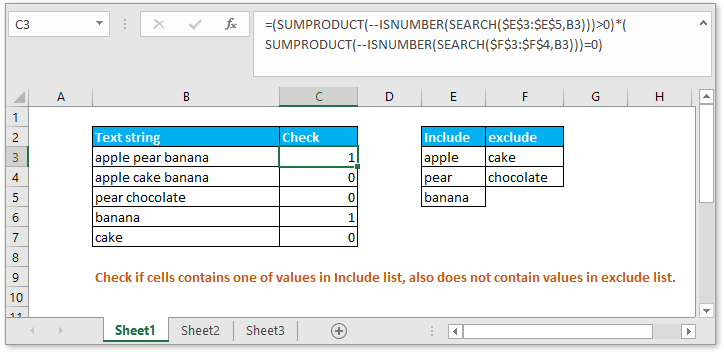Generic formula:

 =(SUMPRODUCT(--ISNUMBER(SEARCH(include,text)))>0) *(SUMPRODUCT(--ISNUMBER(SEARCH(exclude,text)))=0)

Arguments

 Text: the text string you want to check. Include: the values you want to check if argument text contains. Exclude: the values you want to check if argument text does not contain.

Return value:

The formula returns 1 or 0. When the cell contains one of values needed to be included, and does not contain any values needed to be excluded, it returns 1, or it returns 0. This formula, 1 and 0 are handled like logical values TRUE and FALSE.

How this formula work

Supposing you want to check if the cell B3 contains one of values in range E3:E5, but simultaneously exclude values in range F3:F4, please use below formula

 =(SUMPRODUCT(--ISNUMBER(SEARCH(\$E\$3:\$E\$5,B3)))>0)*(SUMPRODUCT(--ISNUMBER(SEARCH(\$F\$3:\$F\$4,B3)))=0)

Press Enter key to get the checking result.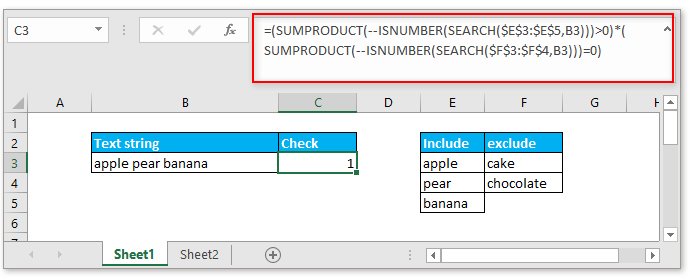Explanation

Part 1: (SUMPRODUCT(--ISNUMBER(SEARCH(\$E\$3:\$E\$5,B3)))>0) checks if cell contains values in E3:E5

SEARCH function: the SEARCH function returns the position of the first character of the text string inside another, if the SEARCH function finds the matched text, it returns the relative position, if not, it returns #VALUE! error. For instance, here the formula SEARCH(\$E\$3:\$E\$5,B3) will search each value of the range E3:E5 in cell B3, and returns the location of each text string in the cell B3. It will return an array result as this: {1;7;12}.

ISNUMBER function: the ISNUMBER function returns TRUE when a cell is a number. So ISNUMBER(SEARCH(\$E\$3:\$E\$5,B3)) will return array result as {true,true,true} as the SEARCH function finds 3 numbers.

--ISNUMBER(SEARCH(\$E\$3:\$E\$5,B3)) converts the TRUE value to 1, and converts FALSE value to 0, so this formula changes the array result to {1;1;1}.

SUMPRODUCT function: is used to multiples ranges or sum arrays together and returns the sum of products. The SUMPRODUCT(--ISNUMBER(SEARCH(\$E\$3:\$E\$5,B3))) returns 1+1+1=3.

At last, compare left formula SUMPRODUCT(--ISNUMBER(SEARCH(\$E\$3:\$E\$5,B3))) and 0, as long as the result of left formula is greater than 0, the result will be TRUE, or it will return FALSE. Here it returns TRUE.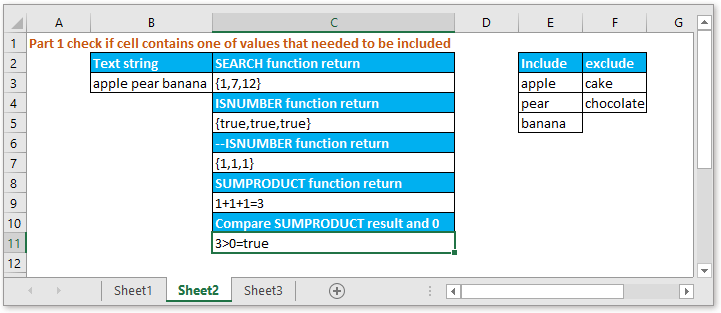Part 2: (SUMPRODUCT(--ISNUMBER(SEARCH(\$F\$3:\$F\$4,B3)))=0) checks if cell does not contain values in F3:F4

The formula SEARCH(\$F\$3:\$F\$4,B3) will search each value in the range E3:E5 in cell B3, and returns the location of each text string in the cell B3. It will return an array result as this: {#VALUE!;#VALUE!}.

ISNUMBER(SEARCH(\$F\$3:\$F\$4,B3)) will return array result as {false;false} as the SEARCH function finds 0 number.

--ISNUMBER(SEARCH(\$F\$3:\$F\$4,B3)) converts the TRUE value to 1, and converts FALSE value to 0, so this formula changes the array result to {0;0 }.

SUMPRODUCT function: is used to multiples ranges or sum arrays together and returns the sum of products. The SUMPRODUCT(--ISNUMBER(SEARCH(\$F\$3:\$F\$4,B3))) returns 0+0=0.

At last, compare left formula SUMPRODUCT(--ISNUMBER(SEARCH(\$F\$3:\$F\$4,B3))) and 0, as long as the result of left formula is equal to 0, the result will return TRUE, or it will return FALSE. Here it returns TRUE.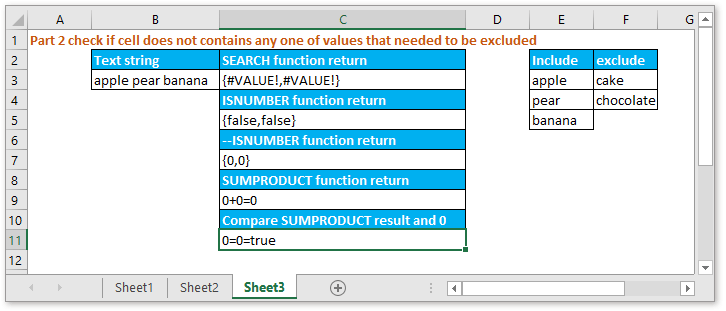Part 3: Multiple two formulas

=(SUMPRODUCT(--ISNUMBER(SEARCH(\$E\$3:\$E\$5,B3)))>0)*(SUMPRODUCT(--ISNUMBER(SEARCH(\$F\$3:\$F\$4,B3)))=0)

=TRUE*TRUE

=1

This formula, 1 and 0 are handled like logical values TRUE and FALSE.

### Sample File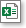Click to download sample file

#### Relative Formulas

• Check if a cell contains a specific text
To check if a cell contains some texts in range A but does not contain the texts in range B, you can use an array formula which combines the COUNT, SEARCH and AND function in Excel
• Check if cell contains one of many things
This tutorial provides a formula to check if a cell contains one of several values in Excel, and explains the arguments in the formula and how the formula work.
• Check if cell contains one of things
Supposing in Excel, there is a list of values in column E, you want to check if the cells in column B contain all of the values in column E, and return TRUE or FALSE.
• Check if cell contains number
Sometimes, you may want to check if a cell contains numeric characters. This tutorial provides a formula which will return TRUE if the cell contains number, FALSE if cell does not contain number.

### The Best Office Productivity Tools

#### Kutools for Excel - Helps You To Stand Out From Crowd

 Popular Features: Find/Highlight/Identify Duplicates  |  Delete Blank Rows  |  Combine Columns or Cells without Losing Data  |  Round without Formula ... Super VLookup: Multiple Criteria  |  Multiple Value   |  Across Multi-Sheets  |  Fuzzy Lookup... Adv. Drop-down List: Easy Drop Down List  |  Dependent Drop Down List  |  Multi-select Drop Down List... Column Manager: Add a Specific Number of Columns  |  Move Columns  |  Unhide Columns  |  Compare Columns to Select Same & Different Cells ... Featured Features: Grid Focus  |  Design View  |  Big Formula Bar  |  Workbook & Sheet Manager  |  Resource Library (Auto Text)  |  Date Picker  |  Combine Worksheets  |  Encrypt/Decrypt Cells  |  Send Emails by List  |  Super Filter  |  Special Filter (filter bold/italic/strikethrough...) ... Top 15 Toolsets:  12 Text Tools (Add Text, Remove Characters ...)  |  50+ Chart Types (Gantt Chart ...)  |  40+ Practical Formulas (Calculate age based on birthday ...)  |  19 Insertion Tools (Insert QR Code, Insert Picture from Path ...)  |  12 Conversion Tools (Numbers to Words, Currency Conversion ...)  |  7 Merge & Split Tools (Advanced Combine Rows, Split Cells ...)  |  ... and more

Kutools for Excel Boasts Over 300 Features, Ensuring That What You Need is Just A Click Away...

Supports Office/Excel 2007-2021 and 365   |   Available in 44 Languages   |   Easy to Uninstall Completely#### Office Tab - Enable Tabbed Reading and Editing in Microsoft Office (include Excel)

• One second to switch between dozens of open documents!
• Reduce hundreds of mouse clicks for you every day, say goodbye to mouse hand.
• Increases your productivity by 50% when viewing and editing multiple documents.
• Brings Efficient Tabs to Office (include Excel), Just Like Chrome, Edge and Firefox.